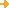search:advanced search

# Screenshot for Two-Variable Linear Equations and Introduction to Functions: Line Graph Intuition

Return to full record for Two-Variable Linear Equations and Introduction to Functions: Line Graph IntuitionReturn to full record for Two-Variable Linear Equations and Introduction to Functions: Line Graph Intuition